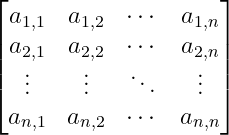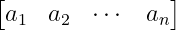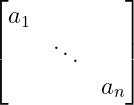# How to denote matrix with dots symbol in LaTeX?

When a large number of rows or columns are used in the matrix. In that case, when denoting the matrix, two to three elements are used and the remaining elements are represented by 3dots symbol.

Notice the table below, where different types of dots symbols have been used. And with that, it has been mentioned where the dots symbols will be located in the matrix.

 Horizontal dots \ldots Vertical dots \vdots Diagonal dots \ddots Inverse Diagonal dots \iddots

## Use dots symbol in n * n square matrix

Three types of dots symbols are used to represent the n * n matrix. For example

\documentclass{article}
\usepackage{amsmath}
\begin{document}
\begin{equation}
\begin{bmatrix}
a_{1,1} & a_{1,2} & \cdots & a_{1,n} \\
a_{2,1} & a_{2,2} & \cdots & a_{2,n} \\
\vdots  & \vdots  & \ddots & \vdots  \\
a_{n,1} & a_{n,2} & \cdots & a_{n,n}
\end{bmatrix}
\end{equation}
\end{document}

Output :## Use vertical dots for row matrix in LaTeX

Since each element of the row matrix is ​​located along the horizontal, horizontal dots are used to represent the elements of the matrix.

\documentclass{article}
\usepackage{amsmath}
\begin{document}
\begin{equation}
\begin{bmatrix}
a_{1} & a_{2} & \cdots & a_{n}\\
\end{bmatrix}
\end{equation}
\end{document}

Output :## Use vertical dots for column matrix in LaTeX

In the case of a column matrix, the elements will be located along a column of the matrix.

\documentclass{article}
\usepackage{amsmath}
\begin{document}
\begin{equation}
\begin{bmatrix}
a_{1} \\ a_{2} \\ \vdots \\ a_{n}
\end{bmatrix}
\end{equation}
\end{document}

Output :## Use diagonal dots for diagonal matrix in LaTeX

In the case of a diagonal matrix, only diagonal dots are used to represent the elements of the matrix along the diagonal.

\documentclass{article}
\usepackage{amsmath}
\begin{document}
\begin{equation}
\begin{bmatrix}
a_{1} &  & \\
& \ddots & \\
&  &  a_{n}
\end{bmatrix}
\end{equation}
\end{document}

Output :And you can use inverse diagonal dots in the matrix especially in cases. For example

\documentclass{article}
\usepackage{amsmath,mathdots}
\begin{document}
\begin{equation}
\begin{bmatrix}
& & a_{1} \\
& \iddots & \\
a_{n} &  &
\end{bmatrix}
\end{equation}
\end{document}

Output :The bmatrix environment has been used to denote the above matrix. This will keep the entire matrix-bound by the square bracket.#### Md Jidan Mondal

LaTeX expert with over 10 years of experience in document preparation and typesetting. Specializes in creating professional documents, reports, and presentations using LaTeX.

### 2 thoughts on “How to denote matrix with dots symbol in LaTeX?”

1.First two examples you begin with {bmatrix} but end with {pmatrix}

1.Thank you very much for the comment. This problem occurred while editing the code. Of course, let’s fix it now.